Image Processing Toolbox User's GuideDiscrete Fourier Transform

Working with the Fourier transform on a computer usually involves a form of the transform known as the discrete Fourier transform (DFT). A discrete transform is a transform whose input and output values are discrete samples, making it convenient for computer manipulation. There are two principal reasons for using this form of the transform:

• The input and output of the DFT are both discrete, which makes it convenient for computer manipulations.
• There is a fast algorithm for computing the DFT known as the fast Fourier transform (FFT).

The DFT is usually defined for a discrete function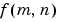that is nonzero only over the finite region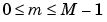and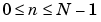. The two-dimensional M-by-N DFT and inverse M-by-N DFT relationships are given by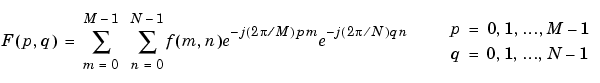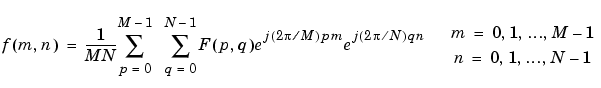The values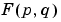are the DFT coefficients of. The zero-frequency coefficient,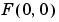, is often called the "DC component." DC is an electrical engineering term that stands for direct current. (Note that matrix indices in MATLAB always start at 1 rather than 0; therefore, the matrix elements `f(1,1)` and `F(1,1)` correspond to the mathematical quantities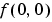and, respectively.)

The MATLAB functions `fft`, `fft2`, and `fftn` implement the fast Fourier transform algorithm for computing the one-dimensional DFT, two-dimensional DFT, and N-dimensional DFT, respectively. The functions `ifft`, `ifft2`, and `ifftn` compute the inverse DFT.

Relationship to the Fourier Transform

The DFT coefficientsare samples of the Fourier transform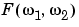.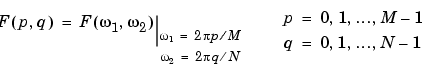Example

1. Construct a matrix `f` that is similar to the function f(m,n) in the example in Definition of Fourier Transform. Remember that f(m,n) is equal to 1 within the rectangular region and 0 elsewhere. Use a binary image to represent f(m,n).
• ```f = zeros(30,30);
f(5:24,13:17) = 1;
imshow(f,'notruesize')```
2. Compute and visualize the 30-by-30 DFT of `f` with these commands.
• ```F = fft2(f);
F2 = log(abs(F));
imshow(F2,[-1 5],'notruesize'); colormap(jet); colorbar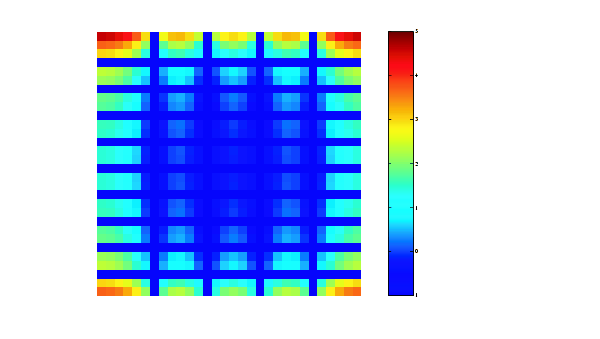```

Discrete Fourier Transform Computed Without Padding

1. This plot differs from the Fourier transform displayed in Visualizing the Fourier Transform. First, the sampling of the Fourier transform is much coarser. Second, the zero-frequency coefficient is displayed in the upper left corner instead of the traditional location in the center.

1. To obtain a finer sampling of the Fourier transform, add zero padding to `f` when computing its DFT. The zero padding and DFT computation can be performed in a single step with this command.
• ```F = fft2(f,256,256);
```
1. This command zero-pads `f` to be 256-by-256 before computing the DFT.

• ```imshow(log(abs(F)),[-1 5]); colormap(jet); colorbar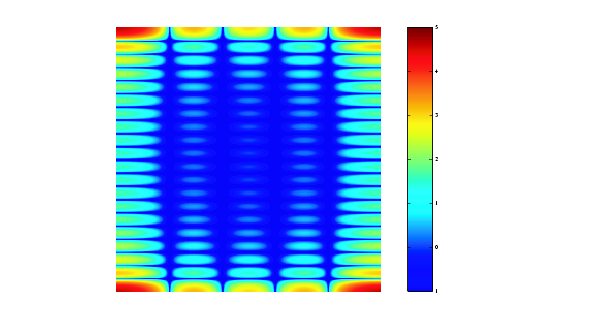```

Discrete Fourier Transform Computed with Padding

1. The zero-frequency coefficient, however, is still displayed in the upper left corner rather than the center. You can fix this problem by using the function `fftshift`, which swaps the quadrants of `F` so that the zero-frequency coefficient is in the center.
• ```F = fft2(f,256,256);
F2 = fftshift(F);
imshow(log(abs(F2)),[-1 5]); colormap(jet); colorbar
```
1. The resulting plot is identical to the one shown in Visualizing the Fourier Transform.Fourier Transform Applications of the Fourier Transform